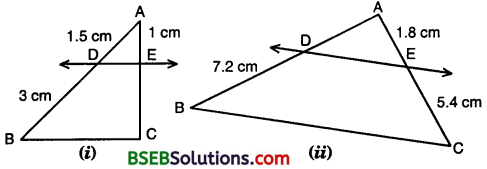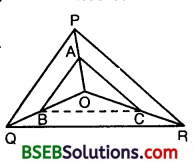# HSSlive: Plus One & Plus Two Notes & Solutions for Kerala State Board

## BSEB Class 10 Maths Chapter 6 Triangles Ex 6.2 Textbook Solutions PDF: Download Bihar Board STD 10th Maths Chapter 6 Triangles Ex 6.2 Book AnswersBSEB Class 10 Maths Chapter 6 Triangles Ex 6.2 Textbook Solutions PDF: Download Bihar Board STD 10th Maths Chapter 6 Triangles Ex 6.2 Book Answers

BSEB Class 10th Maths Chapter 6 Triangles Ex 6.2 Textbooks Solutions and answers for students are now available in pdf format. Bihar Board Class 10th Maths Chapter 6 Triangles Ex 6.2 Book answers and solutions are one of the most important study materials for any student. The Bihar Board Class 10th Maths Chapter 6 Triangles Ex 6.2 books are published by the Bihar Board Publishers. These Bihar Board Class 10th Maths Chapter 6 Triangles Ex 6.2 textbooks are prepared by a group of expert faculty members. Students can download these BSEB STD 10th Maths Chapter 6 Triangles Ex 6.2 book solutions pdf online from this page.

## Bihar Board Class 10th Maths Chapter 6 Triangles Ex 6.2 Books Solutions

 Board BSEB Materials Textbook Solutions/Guide Format DOC/PDF Class 10th Subject Maths Chapter 6 Triangles Ex 6.2 Chapters All Provider Hsslive

## How to download Bihar Board Class 10th Maths Chapter 6 Triangles Ex 6.2 Textbook Solutions Answers PDF Online?

2. Click on the Bihar Board Class 10th Maths Chapter 6 Triangles Ex 6.2 Answers.
3. Look for your Bihar Board STD 10th Maths Chapter 6 Triangles Ex 6.2 Textbooks PDF.
4. Now download or read the Bihar Board Class 10th Maths Chapter 6 Triangles Ex 6.2 Textbook Solutions for PDF Free.

Find below the list of all BSEB Class 10th Maths Chapter 6 Triangles Ex 6.2 Textbook Solutions for PDF’s for you to download and prepare for the upcoming exams:

## BSEB Bihar Board Class 10th Maths Solutions Chapter 6 Triangles Ex 6.2

Question 1.
In figures, (i) and (ii), DE || BC. Find EC in (i) and AD in (ii).Solution:
(i) In Fig. (i),
since DE || BC, therefore
𝐴𝐷𝐷𝐵 = 𝐴𝐸𝐸𝐶 or 1.53 = 1𝐸𝐶
or EC = 31.5 = 3×1015 Cm = 2 cm

(ii) In Fig. (ii),
since DE || BC, therefore
𝐴𝐷𝐷𝐵 =𝐴𝐸𝐸𝐶 or 𝐴𝐷7.2 = 1.85.4
or AD = 1854 x 7210 cm = 2.4 cm.

Question 2.
E and F are points on the sides PQ and PR respectively of a A PQR. For each of the following cases, state whether EF || QR :
(i) PE = 3.9 cm, EQ = 3 cm, PF = 3.6 cm and FR = 2.4 cm
(ii) PE = 4 cm, QE = 4.5 cm, PF = 8 cm and RF = 9 cm
(iii) PQ = 1.28 cm, PR = 2.56 cm, PE = 0.18 cm and PF = 0.36 cm
Solution:
(i) We have :
PE = 3.9 cm, EQ = 3 cm,
PF = 3.6 cm and FR = 2.4 cmi. e., EF does not divide the sides PQ and PR of A PQR in the same ratio. Therefore, EF is not parallel to QR.

(ii) We have : PE = 4 cm, QE = 4.5 cm, PF = 8 cm and RF = 9 cm
Now, 𝑃𝐸𝐸𝑄 = 44.5 = 4045 = 89
and 𝑃𝐹𝐹𝑅 = 89
So, 𝑃𝐸𝐸𝑄 = 𝑃𝐹𝐹𝑅
Thus, EF divides sides PQ and PR of ∆ PQR in the same ratio. Therefore, by the converse of Basic Proportionality Theorem, we have EF || QR.

(iii) We have :PQ= 1.28 cm, PR = 2.56 cm,
PE = 0.18 cm and PF 0.36 cm
∴ EQ = PQ – PE = (1.28 – 0.18) cm = 1.10 cm
and FR = PR – PF = (2.56 – 0.36) = 2.20 cm
Now, 𝑃𝐸𝐸𝑄 = 0.181.10 = 18110 = 955
and 𝑃𝐹𝐹𝑅 = 0.362.20 = 36220 = 955
So, 𝑃𝐸𝐸𝑄 = 𝑃𝐹𝐹𝑅
Thus, EF divides sides PQ and PR of ∆ PQR in the same ratio. Therefore, by the converse of Basic Proportionality Theorem, we have EF || QR.

Question 3.
In the figure, if LM || CB and LN || CD, prove that 𝐴𝑀𝐴𝐵 = 𝐴𝑁𝐴𝐷Solution:
In ∆ ABC, we have :
LM || CB
∴By a result based on Basic Proportionality Theorem (a corollary), we have :
𝐴𝑀𝐴𝐵 = 𝐴𝐿𝐴𝐶 … (1)
In ∆ ACD, we have :
LN || CD [Given]
∴By a result based on Basic Proportionality Theorem, we have :
𝐴𝐿𝐴𝐶 = 𝐴𝑁𝐴𝐷
From (1) and (2), we obtain that
𝐴𝑀𝐴𝐵 = 𝐴𝑁𝐴𝐷

Question 4.
In the figure, DE || AC and DF || AE. Prove that 𝐵𝐹𝐹𝐸 = 𝐵𝐸𝐸𝐶Solution:
In ∆ BGA, we have :
DE || ‘AC
∴By Basic Proportionality Theorem, we have :
𝐵𝐸𝐸𝐶 = 𝐵𝐷𝐷𝐴 …. (1)
In ∆ BEA, we have :
DF || AE [Given]
∴By Basic Proportionality Theorem, we have
𝐵𝐹𝐹𝐸 = 𝐵𝐷𝐷𝐴 …. (2)
From (1) and (2), we obtain that
𝐵𝐹𝐹𝐸 = 𝐵𝐸𝐸𝐶.

Question 5.
In the figure, DE || OQ and DF || OR. Show that EF || QR.Solution:
In ∆ PQO, we have :
DE || OQ [Given]
∴By Basic Proportionality Theorem, we have :
𝑃𝐸𝐸𝑄 = 𝑃𝐷𝐷𝑂 … (1)
In ∆ POR, We have :
DF || OR [Given]
∴By Basic Proportionality Theorem, we have
𝑃𝐷𝐷𝑂 = 𝑃𝐹𝐹𝑅 … (2)
From (1) and (2), we obtain that
𝑃𝐸𝐸𝑄 = 𝑃𝐹𝐹𝑅
So, EF || QR [By the converse of BPT]

Question 6.
In the figure, A, B and C are points on OP, OQ and OR respectively such that AB || PQ and AC || PR. Show that BC || QR.Solution:
Given : O is any point within ∆ PQR, AB || PQ and AC || PR
To prove : BC || QR.
Construction : Join BC.
Proof:
In ∆ OPQ, we have :
AB || PQ [Given]
∴By Basic Proportionality Theorem, we have :
𝑂𝐴𝐴𝑃 = 𝑂𝐵𝐵𝑄 … (1)
In ∆ OPR, We have :
AC || PR [Given]
∴ By Basic Proportionality Theorem, we have
𝑂𝐴𝐴𝑃 = 𝑂𝐶𝐶𝑅 … (2)
From (1) and (2), we obtain that
𝑂𝐵𝐵𝑄 = 𝑂𝐶𝐶𝑅
Thus, in ∆ OQR, B and C are points dividing the sides OQ and OR in the same ratio. Therefore, by the converse of Basic Proportionality Theorem, we have :
BC || QR.

Question 7.
Using Theorem 6.1, prove that a line drawn through the mid-point of one side of a triangle parallel to another side bisects the third side. (Recall that you have proved it in Class IX).Solution:
Given : ∆ ABC, in which D is the mid-point of side AB and the line DE is drawn parallel to BC, meeting AC in E.
To prove : AE = EC
Proof:
Since DE || BC, therefore by Basic Proportionality Theorem, we have :
𝐴𝐷𝐷𝐵 = 𝐴𝐸𝐸𝐶 … (1)
But AD = DB [∵D is the mid-point of AB]
i.e., 𝐴𝐷𝐷𝐵 = 1
∴ From (1), 𝐴𝐸𝐸𝐶 = 1 or AE = EC
Hence, E is the mid-point of the third side AC.

Question 8.
Using Theorem 6.2, prove that the line joining the mid-points of any two sides of a triangle is parallel to the third side. (Recall that you have done it in Class IX).Solution:
Given : ∆ ABC, in which D and E are the mid¬points of sides AB and AC respectively.
To prove : DE || BC.
Proof :
Since D and E are the mid-points of AB and AC respectively, therefore
AD = DB and AE = EC
∴ 𝐴𝐷𝐷𝐵 = 1
and AE = EC
∴ 𝐴𝐸𝐸𝐶 = 1
Thus, in ∆ ABC, D and E are points dividing the sides AB and AC in the same ratio. Therefore, by the converse of Basic Proportionality Theorem (Theorem 6.2), we have :
DE || BC.

Question 9.
ABCD is a trapezium in which AB || DC and its diagonals intersect each other at the point O. Show that 𝐴𝑂𝐵𝑂 = 𝐶𝑂𝐷𝑂.
Solution:
Given : A trapezium ABCD, in which AB || DC and its diagonals AC and BD intersect each other at O.
To prove : 𝐴𝑂𝐵𝑂 = 𝐶𝑂𝐷𝑂.
Construction : Through O, draw OE || AB, i.e., OE || DC.
Proof:
In ∆ ADC, we have :
OE || DC [Construction]
∴ By Basic Proportionality Theorem, we have :𝐴𝐸𝐸𝐷 = 𝐴𝑂𝐶𝑂 … (1)
Again, in ∆ ABD, we have :
OE || AB [Construction]
∴By Basic Proportionality Theorem, we have :0
𝐸𝐷𝐴𝐸 = 𝐷𝑂𝐵𝑂 or 𝐴𝐸𝐸𝐷 = 𝐵𝑂𝐷𝑂 … (2)
From (1) and (2), we obtain that
𝐴𝑂𝐶𝑂 = 𝐵𝑂𝐷𝑂 or 𝐴𝑂𝐵𝑂 = 𝐶𝑂𝐷𝑂.

Question 10.
The diagonals of a quadrilateral ABCD intersect each other at the point O such that 𝐴𝑂𝐵𝑂 = 𝐶𝑂𝐷𝑂 that ABCD is a trapezium.
Solution:
Given : A quadrilateral ABCD in which its diagonals AC and BD intersect each other at the point O such that 𝐴𝑂𝐵𝑂 = 𝐶𝑂𝐷𝑂, i.e., 𝐴𝑂𝐶𝑂 = 𝐵𝑂𝐷𝑂
To prove : Quadrilateral ABCD is a trapezium.
Construction : Through O, draw OE || AB meeting AD in E.
Proof:
In ∆ ADB, we have :
OE || AB [Construction]
∴ By Basic Proportionality Theorem, we have :
𝐷𝐸𝐸𝐴 = 𝑂𝐷𝐵𝑂
or 𝐸𝐴𝐷𝐸 = 𝐵𝑂𝐷𝑂
i.e., 𝐸𝐴𝐷𝐸 = 𝐵𝑂𝐷𝑂 = 𝐴𝑂𝐶𝑂 [ ∵𝐴𝑂𝐶𝑂 = 𝐵𝑂𝐷𝑂(given)]
or 𝐸𝐴𝐷𝐸 = 𝐴𝑂𝐶𝑂
Thus, in ∆ ADC, points E and O are dividing the sides AD and AC in the same ratio. Therefore, by the converse of Basic Proportionality Theorem, we have :
EO || DC
But, EO || AB [construction]
Hence, AB || DC
∴ Quadrilateral ABCD is a trapezium

## Bihar Board Class 10th Maths Chapter 6 Triangles Ex 6.2 Textbooks for Exam Preparations

Bihar Board Class 10th Maths Chapter 6 Triangles Ex 6.2 Textbook Solutions can be of great help in your Bihar Board Class 10th Maths Chapter 6 Triangles Ex 6.2 exam preparation. The BSEB STD 10th Maths Chapter 6 Triangles Ex 6.2 Textbooks study material, used with the English medium textbooks, can help you complete the entire Class 10th Maths Chapter 6 Triangles Ex 6.2 Books State Board syllabus with maximum efficiency.

## FAQs Regarding Bihar Board Class 10th Maths Chapter 6 Triangles Ex 6.2 Textbook Solutions

#### Can we get a Bihar Board Book PDF for all Classes?

Yes you can get Bihar Board Text Book PDF for all classes using the links provided in the above article.

## Important Terms

Bihar Board Class 10th Maths Chapter 6 Triangles Ex 6.2, BSEB Class 10th Maths Chapter 6 Triangles Ex 6.2 Textbooks, Bihar Board Class 10th Maths Chapter 6 Triangles Ex 6.2, Bihar Board Class 10th Maths Chapter 6 Triangles Ex 6.2 Textbook solutions, BSEB Class 10th Maths Chapter 6 Triangles Ex 6.2 Textbooks Solutions, Bihar Board STD 10th Maths Chapter 6 Triangles Ex 6.2, BSEB STD 10th Maths Chapter 6 Triangles Ex 6.2 Textbooks, Bihar Board STD 10th Maths Chapter 6 Triangles Ex 6.2, Bihar Board STD 10th Maths Chapter 6 Triangles Ex 6.2 Textbook solutions, BSEB STD 10th Maths Chapter 6 Triangles Ex 6.2 Textbooks Solutions,
Share: